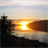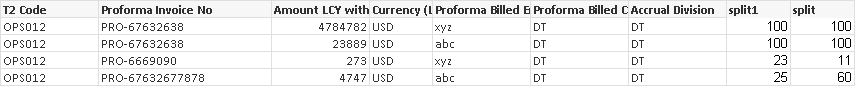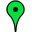# QlikView App Dev

Discussion Board for collaboration related to QlikView App Development.

Announcements
Support Cases coming to Qlik Community Oct. 4! Start chats, open cases, explore resources: READ DETAILS
cancel
Showing results for
Did you mean:Creator II

## How to write if condition

Hi,

how to fulfil two condition in same expression.

This is the table attached below.Expression which I am using for Amount LCY with split column is:-

=if(ProformaParent<>'NULL' and Split1=split ,sum(split*total_amount_fct)/100 ,

if(ProformaParent<>'NULL' and Split1<>split ,sum(split*total_amount_fct)/100 )

Requirement is if split1=split then this formula sum(split*total_amount_fct)/100 should apply, otherwise if split1<>split

then sum(split*total_amount_fct)/100 formula should be applicable.

I tried to do that, but at a time only one condition is fulfilling.

1 Solution

Accepted SolutionsMVP

For both the condition expressions is same (sum(split*total_amount_fct)/100)? try below

if(ProformaParent<>'NULL' and (Split1=split or Split1<>split), sum(split*total_amount_fct)/100)

7 RepliesMVP

For both the condition expressions is same (sum(split*total_amount_fct)/100)? try below

if(ProformaParent<>'NULL' and (Split1=split or Split1<>split), sum(split*total_amount_fct)/100)Specialist III

SUM(IF(Not(IsNull(ProformaParent)),IF(split1<>split,sum(split1*total_amount_fct)/100,sum(split*total_amount_fct)/100))

RegardsCreator II

Hi Shikha,

if(ProformaParent<>NULL() and Split1=split ,sum(split*total_amount_fct)/100 ,

if(ProformaParent<>NULL() and Split1<>split ,sum(split*total_amount_fct)/100 ))

Regards,

SKSpecialist III

Sorry, my last expression is wrong:

SUM(IF(Not(IsNull(ProformaParent)),IF(split1<>split,split1*total_amount_fct/100,split*total_amount_fct/100)))

We put IF conditions into sum expression to calculate the correct result on total rows.

RegardsSpecialist

Hi

try this

if(not isnull(ProformaParent) and Split1=split ,sum(split*total_amount_fct)/100 ,

if(not isnull(ProformaParent) and Split1<>split ,sum(split*total_amount_fct)/100 ))Creator

Why do you need Split1 comparison condition, if you expect to display same expression in both the cases ?Partner

Indeed. The solution to your current post doesn't need two conditions. It can be resolved using this expression:

=IF (NOT IsNull(ProformaParent), Sum(split * total_amount_fct) / 100)

Can you rewrite your OP to make it more clear what you're after? Thanks.

PeterCommunity Browser